Journal topic
Solid Earth, 11, 855–871, 2020
https://doi.org/10.5194/se-11-855-2020Solid Earth, 11, 855–871, 2020
https://doi.org/10.5194/se-11-855-2020Research article 08 May 2020

Research article | 08 May 2020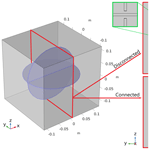# Azimuth-, angle- and frequency-dependent seismic velocities of cracked rocks due to squirt flow

Azimuth-, angle- and frequency-dependent seismic velocities of cracked rocks due to squirt flow
Yury Alkhimenkov1,2, Eva Caspari3, Simon Lissa1, and Beatriz Quintal1,2 Yury Alkhimenkov et al.
• 1Institute of Earth Sciences, University of Lausanne, Lausanne, Switzerland
• 2Swiss Geocomputing Centre, University of Lausanne, Lausanne, Switzerland
• 3Chair of Applied Geophysics, Montanuniversity Leoben, Leoben, Austria

Correspondence: Yury Alkhimenkov (yury.alkhimenkov@unil.ch)

Abstract

Understanding the properties of cracked rocks is of great importance in scenarios involving CO2 geological sequestration, nuclear waste disposal, geothermal energy, and hydrocarbon exploration and production. Developing noninvasive detecting and monitoring methods for such geological formations is crucial. Many studies show that seismic waves exhibit strong dispersion and attenuation across a broad frequency range due to fluid flow at the pore scale known as squirt flow. Nevertheless, how and to what extent squirt flow affects seismic waves is still a matter of investigation. To fully understand its angle- and frequency-dependent behavior for specific geometries, appropriate numerical simulations are needed. We perform a three-dimensional numerical study of the fluid–solid deformation at the pore scale based on coupled Lamé–Navier and Navier–Stokes linear quasistatic equations. We show that seismic wave velocities exhibit strong azimuth-, angle- and frequency-dependent behavior due to squirt flow between interconnected cracks. Furthermore, the overall anisotropy of a medium mainly increases due to squirt flow, but in some specific planes the anisotropy can locally decrease. We analyze the Thomsen-type anisotropic parameters and adopt another scalar parameter which can be used to measure the anisotropy strength of a model with any elastic symmetry. This work significantly clarifies the impact of squirt flow on seismic wave anisotropy in three dimensions and can potentially be used to improve the geophysical monitoring and surveying of fluid-filled cracked porous zones in the subsurface.

1 Introduction

Wave propagation is controlled by the effective rock properties. Wave velocity and attenuation can be estimated from seismic data in scenarios such as seismic exploration, seismology, borehole measurements and tomography. Rock physics could then be used to estimate different rock properties, such as mineral composition, elastic moduli, the presence of a fluid, and pore space connectivity (and hence permeability) from seismic measurements. Thus, investigation of how cracks and fluids affect seismic properties has many practical applications. In activities including nuclear waste disposal, CO2 geological sequestration, hydrocarbon exploration and production, geothermal energy production, and seismotectonics, a quantification of the fluid content, porosity and permeability of rocks are of great interest. All these activities can benefit from rock physics studies, and that is why cracked rocks have been under intensive studies during the last decades.

Cracks and grain-scale discontinuities are the key rock parameters which control effective elastic and hydraulic properties of such rocks. Many studies show that seismic waves exhibit significant dispersion and attenuation in cracked porous rocks due to pore-scale fluid flow . Furthermore, cracks cause significant seismic wave anisotropy .

Fluid flow due to a passing wave may happen at different scales: at the wavelength scale, at the mesoscopic scale and at the pore scale . Biot's theory (Biot1962) describes the so-called global flow at the wavelength scale, but its overall effect on a passing wave at seismic frequencies is usually much smaller than that of fluid flow at the mesoscopic and pore scales . The mesoscopic scale is that much larger than the pore-scale but smaller than the wavelength. At this scale, studies are performed in the framework of Biot theory, assuming heterogeneous rock properties. One can define fractures as discontinuities at the mesoscopic scale and cracks as discontinuities at the pore scale. There are several analytical and numerical studies on the effect of wave-induced fluid flow between mesoscopic fractures and a porous rock background and between interconnected fractures using Biot's equations as well as on the comparison between the numerical and analytical results . Experimental studies of synthetic rock samples showed the impact of fluid-saturated fractures on seismic velocities . The resulting frequency-dependent anisotropy was analyzed by , and . The last two also considered fracture-to-fracture flow, in addition to fracture-to-background flow.

At the pore scale, a passing wave induces fluid pressure gradients which occur between interconnected cracks, as well as between cracks and stiffer pores. Such pressure gradients force fluid to move between different cracks and pores until the pore pressure equilibrates throughout the connected pore space. This phenomenon, known as squirt flow , causes strong energy dissipation due to the viscosity of the fluid and the associated viscous friction. Several experimental studies confirmed the importance of squirt flow at different frequency ranges . There are several analytical solutions for squirt flow , which are based on simplified pore geometries and many physical assumptions.

Dispersion and attenuation caused by squirt flow can be simulated numerically by solving the coupled fluid–solid deformation at the pore scale using Lamé–Navier and Navier–Stokes equations with appropriate boundary conditions and then calculating effective frequency-dependent viscoelastic properties. During the last decades, many studies used numerical methods to solve mechanical problems . Recently, some numerical studies appeared in the geophysical literature aiming to solve the coupled fluid–solid deformation and hence studying dispersion and attenuation caused by squirt flow . numerically simulated a fully coupled fluid–solid interaction at the pore scale for digital rock samples. They modeled the pore fluids as Newtonian fluids using the Navier–Stokes equation with appropriate coupling between both the solid and liquid phases, accounting for inertial effects. simplified the Navier–Stokes equations by neglecting the inertial term and hence used the linearized quasistatic Navier–Stokes equation.

We numerically simulate squirt flow in three dimensions and calculate frequency-dependent effective stiffness moduli using the finite-element method to solve the quasistatic Lamé–Navier equations coupled to the linearized quasistatic Navier–Stokes equations . We apply an oscillatory deformation to certain boundaries of the numerical model, and, assuming that the wavelength is much larger than the size of individual cracks, we calculate the volume-average stress and strain fields and the resulting effective stiffness moduli. Then, we calculate the associated azimuth-, angle- and frequency-dependent seismic velocities by solving the Christoffel equation. The main goal of this study is to analyze seismic anisotropy due to squirt flow in three dimensions since the previous numerical studies of seismic anisotropy were performed only in two dimensions and in the framework of Biot's theory .

This paper is organized as follows. First, we briefly describe the numerical methodology. Then, we describe the numerical model and show the numerical results – frequency-dependent effective stiffness moduli. After, by solving the Christoffel equation, we evaluate the angle-, azimuth- and frequency dependent velocities of the model. Lastly, we quantify the anisotropy strength of the models analyzing the conventional Thomsen-type anisotropy parameters and also by adopting another scalar parameter.

2 Numerical methodology

We consider that at the pore scale, a rock is composed of a solid material (grains) and a fluid-saturated pore space (cracks). The numerical methodology is described by and , and here we briefly outline the main equations. The solid phase is described as a linear isotropic elastic material for which the conservation of momentum is (e.g., and )

$\begin{array}{}\text{(1)}& \mathrm{\nabla }\cdot \mathbit{\sigma }=\mathrm{0},\end{array}$

where “∇⋅” denotes the divergence operator acting on the stress tensor σ. The infinitesimal stress–strain relation for an elastic material can be written as

$\begin{array}{}\text{(2)}& \begin{array}{rl}\mathbit{\sigma }=& \left(K-\frac{\mathrm{2}}{\mathrm{3}}\mathit{\mu }\right)\text{tr}\left(\frac{\mathrm{1}}{\mathrm{2}}\left(\left(\mathrm{\nabla }\otimes \mathbit{u}\right)+\left(\mathrm{\nabla }\otimes \mathbit{u}{\right)}^{\text{T}}\right)\right){\mathbf{I}}_{\mathrm{2}}\\ +& \mathrm{2}\mathit{\mu }\left(\frac{\mathrm{1}}{\mathrm{2}}\left(\left(\mathrm{\nabla }\otimes \mathbit{u}\right)+\left(\mathrm{\nabla }\otimes \mathbit{u}{\right)}^{\text{T}}\right)\right),\end{array}\end{array}$

where I2 is the second-order identity tensor, tr is the trace operator, “” defines the tensor product, the superscript “T” corresponds to the transpose operator, u is the displacement vector, and K and μ are respectively the bulk and shear moduli.

The fluid phase is described by the quasistatic linearized compressible Navier–Stokes momentum equation :

$\begin{array}{}\text{(3)}& -\mathrm{\nabla }p+\mathit{\eta }{\mathrm{\nabla }}^{\mathrm{2}}\mathbit{v}+\frac{\mathrm{1}}{\mathrm{3}}\mathit{\eta }\mathrm{\nabla }\left(\mathrm{\nabla }\cdot \mathbit{v}\right)=\mathrm{0},\end{array}$

where v is the particle velocity, p is the fluid pressure and η is the shear viscosity. Equation (3) is valid for the laminar flow of a Newtonian fluid. In the finite-element numerical solver, Eqs. (2)–(3) are combined in the space–frequency domain

$\begin{array}{}\text{(4)}& {\mathit{\sigma }}_{ij}=\mathit{\lambda }e{\mathit{\delta }}_{ij}+\mathrm{2}\mathit{\mu }{\mathit{ϵ}}_{ij}+i\mathit{\omega }\left(\mathrm{2}\mathit{\eta }{\mathit{ϵ}}_{ij}-\frac{\mathrm{2}}{\mathrm{3}}\mathit{\eta }e{\mathit{\delta }}_{ij}\right),\end{array}$

where ϵij represents the components of the strain tensor ${\mathit{ϵ}}_{ij}=\mathrm{0.5}\left({u}_{i,j}+{u}_{j,i}\right)$, e is the trace of the strain tensor, λ and μ are the Lame parameters, ui is the displacement in the ith direction, δij is the Kronecker delta, i is the imaginary unit, and ω is the angular frequency. In the domain representing a solid material, Eq. (4) reduces to Eq. (2) by setting the shear viscosity η to zero. In the domain representing compressible viscous fluid, Eq. (3) is recovered by setting the shear modulus μ to zero. The solid and fluid displacements are described by the same variable, and thus they are naturally coupled at the boundaries between subdomains . In the simulations, the energy dissipation is caused only by fluid pressure diffusion, since inertial terms are neglected.

The COMSOL Multiphysics partial differential equation module is used for implementing Eqs. (1) and (4) (displacement-stress formulation) in a weak form. Our numerical results can be fully reproduced by using any open-access software which includes mesh generation and finite-element implementation with a corresponding solver for a linear system of equations. The whole spatial domain is discretized using an unstructured mesh with tetrahedral elements. A direct PARDISO solver is used for solving the linear system of equations. Direct relaxation tests are performed to compute all components of the stiffness matrix (in Voigt notation) cij. The basic idea of the direct relaxation tests is that a displacement boundary condition of the form $u={\mathrm{10}}^{-\mathrm{6}}×\mathrm{exp}\left(i\mathit{\omega }t\right)$ is applied to a certain external wall of the model and in a certain direction, while at other walls of the model the displacements are set to zero or let free to change. In the direct tests that we perform, only one component of the stiffness matrix cij can be directly calculated after one numerical simulation. A detailed description of the boundary conditions is given in . The initial conditions for displacements are set to zero. The resulting stress and strains are averaged over the spatial domain for each frequency. Then, the complex-valued cii(ω) components (diagonal) are calculated for each frequency (in Voigt notation, no index summation):

$\begin{array}{}\text{(5)}& {c}_{ii}\left(\mathit{\omega }\right)=\frac{〈{\mathit{\sigma }}_{i}\left(\mathit{\omega }\right)〉}{〈{\mathit{ϵ}}_{i}\left(\mathit{\omega }\right)〉},\end{array}$

where 〈⋅〉 represents the volume averaging over the sample volume. For calculating the P-wave modulus ($ii=\mathrm{11},\mathrm{22},\mathrm{33}$), a harmonic displacement on the i direction is applied perpendicularly to a wall of the model. At the other walls of the model, the normal component of the displacement is set to zero. For calculating shear components of the stiffness matrix ($ii=\mathrm{44},\mathrm{55},\mathrm{66}$), the boundary conditions applied are those of a simple shear test. For the c12(ω), c13(ω) and c23(ω) components (off-diagonal), mixed direct tests are needed, and the corresponding boundary conditions are given in Appendix A. The corresponding inverse quality factor is

$\begin{array}{}\text{(6)}& \frac{\mathrm{1}}{{Q}_{ij}\left(\mathit{\omega }\right)}=\frac{\text{Im}\left({c}_{ij}\left(\mathit{\omega }\right)\right)}{\text{Re}\left({c}_{ij}\left(\mathit{\omega }\right)\right)}.\end{array}$

Note that usually the inverse quality factor is used as a measure of attenuation . In this study, we show the inverse quality factor for each component of the stiffness tensor, even though the ratio Im(cij(ω))∕Re(cij(ω)) does not represent attenuation of any corresponding wave mode for some components.

3 Numerical model

Two 3D numerical models are constructed, which consist of a pore space embedded into an elastic solid grain material (Fig. 1). The solid grain material is represented by a cuboid whose size is ($\mathrm{0.24}×\mathrm{0.24}×\mathrm{0.24}$) m3. The pore space consists of two perpendicular cracks represented by thin cylinders of 0.002 m thickness, 0.1 m radius (i.e., the aspect ratio is thickness divided by diameter – 0.01) and fully saturated with a liquid. In the first model, the two cracks are disconnected, while, in the second model, the two cracks are connected (cross sections in Fig. 1). The employed liquid properties are those of glycerol, and the grain material has properties of quartz (Table 1).

A fine, regular mesh is used inside the crack to accurately account for dissipation, while in the grain material the mesh is coarser (Fig. 2). The total number of elements is 3.3×106. The simulation is performed for 12 different frequencies from 101 to 106.5 Hz for each of the nine components of the stiffness matrix (c11, c22, c33, c12, c13, c23, c44, c55, c66). For each frequency, the solver uses approximately 0.95 TB (terabytes) of RAM memory and takes approximately 2.5 h on 32 Intel dual-socket E5-2683 v4 2.1 GHz (1024 GB RAM) cores.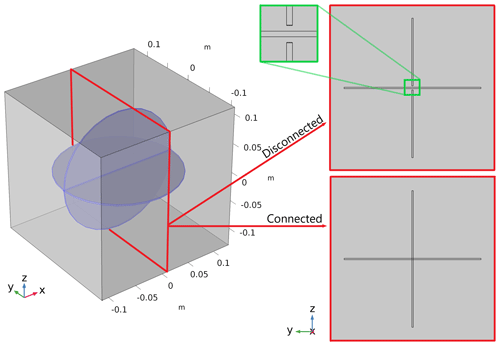Figure 1Sketch illustrating two flat cylinders representing two cracks. The blue region represents the pore space saturated with a fluid and the transparent gray area corresponds to the solid grain material. In the first model, the two cracks are disconnected as illustrated by the upper right sketch. In the second model, the two cracks are connected as illustrated by the lower right sketch.

One crack embedded into an isotropic background induces a transverse isotropy (five independent components of the stiffness tensor, e.g., ). If the crack is parallel to the xy plane, then the symmetry is vertical and the medium exhibits vertical transverse isotropy – VTI symmetry. If the crack is parallel to the xz plane, then the symmetry is horizontal and the medium exhibits horizontal transverse isotropy – HTI symmetry. If two cracks, perpendicular to each other, are embedded into an isotropic material and the crack compliances are different, then the medium exhibits orthorhombic symmetry (nine independent components of the stiffness tensor). If the crack compliances are the same, then the medium symmetry is tetragonal (six independent components of the stiffness tensor); some authors attribute this geometry to a special case of orthorhombic symmetry (e.g., ), while tetragonal and orthorhombic symmetry classes are different. On the other hand, one can argue that an orthorhombic medium (created by two perpendicular sets of cracks) degenerates into a tetragonal medium if the crack compliances are the same.

The symmetry of the saturated numerical model with connected cracks is tetragonal (Fig. 1), because the crack compliances are the same. Thus, there are only six independent components of the stiffness tensor. We will see that the symmetry of the saturated numerical model with disconnected cracks is orthorhombic, because one crack is stiffer than the other one due to its separation into two parts. However, the difference between c22 and c33 stiffness components is less then 0.3 %, thus the divergence from the tetragonal symmetry is negligible, and therefore this model is considered tetragonal as well.

4 Results

## 4.1 Dry stiffness moduli

Let us first consider the geometry shown in Fig. 1 with a pore space filled with air (i.e., dry). We perform nine relaxation tests to calculate the full stiffness tensor for each of the two models with connected and disconnected cracks. The resulting effective stiffness moduli for the model with connected cracks are (in Voigt notation)

$\begin{array}{}\text{(7)}& {c}_{ij}^{\text{Con}}=\left[\begin{array}{cccccc}\mathrm{93.53}& \mathrm{4.65}& \mathrm{4.65}& \mathrm{0}& \mathrm{0}& \mathrm{0}\\ \mathrm{4.65}& \mathrm{63.91}& \mathrm{5.46}& \mathrm{0}& \mathrm{0}& \mathrm{0}\\ \mathrm{4.65}& \mathrm{5.46}& \mathrm{63.91}& \mathrm{0}& \mathrm{0}& \mathrm{0}\\ \mathrm{0}& \mathrm{0}& \mathrm{0}& \mathrm{31.62}& \mathrm{0}& \mathrm{0}\\ \mathrm{0}& \mathrm{0}& \mathrm{0}& \mathrm{0}& \mathrm{35.16}& \mathrm{0}\\ \mathrm{0}& \mathrm{0}& \mathrm{0}& \mathrm{0}& \mathrm{0}& \mathrm{35.16}\end{array}\right]\left(\text{GPa}\right).\end{array}$

For the model with disconnected cracks, the effective stiffness moduli are (in Voigt notation)

$\begin{array}{}\text{(8)}& {c}_{ij}^{\text{Dis}}=\left[\begin{array}{cccccc}\mathrm{93.55}& \mathrm{4.92}& \mathrm{4.60}& \mathrm{0}& \mathrm{0}& \mathrm{0}\\ \mathrm{4.92}& \mathrm{69.21}& \mathrm{4.40}& \mathrm{0}& \mathrm{0}& \mathrm{0}\\ \mathrm{4.60}& \mathrm{4.40}& \mathrm{64.06}& \mathrm{0}& \mathrm{0}& \mathrm{0}\\ \mathrm{0}& \mathrm{0}& \mathrm{0}& \mathrm{31.95}& \mathrm{0}& \mathrm{0}\\ \mathrm{0}& \mathrm{0}& \mathrm{0}& \mathrm{0}& \mathrm{35.16}& \mathrm{0}\\ \mathrm{0}& \mathrm{0}& \mathrm{0}& \mathrm{0}& \mathrm{0}& \mathrm{36.96}\end{array}\right]\left(\text{GPa}\right).\end{array}$

The effective stiffness moduli of the two models are different. Zero values are written if the value is below 0.0002 GPa (i.e., up to numerical precision). The ${c}_{ij}^{\text{Con}}$ stiffness matrix precisely belongs to the tetragonal symmetry class, while the ${c}_{ij}^{\text{Dis}}$ stiffness matrix has all diagonal components different from each other; thus, it represents the orthorhombic symmetry class. The largest difference between ${c}_{ij}^{\text{Con}}$ and ${c}_{ij}^{\text{Dis}}$ is in the c22 component; i.e., $\mathrm{\Delta }{c}_{\mathrm{22}}={c}_{\mathrm{22}}^{\text{Dis}}-{c}_{\mathrm{22}}^{\text{Con}}=\mathrm{5.3}$ GPa. That is a significant difference and it is only due to the vertical crack separation. There are two different features which must be clearly separated. (1) The effect of crack intersection without changing the crack geometry on the effective elastic properties. In this case, the crack intersection is achieved by changing the spatial position of the cracks. studied numerically the effect of crack intersection without changing the crack geometry. They concluded that crack intersection has a very little impact on the effective elastic moduli. (2) The effect of the crack partition into two “halves” on the effective elastic properties. In this case, the partitioned crack has a long, thin contact area across the whole diameter (Fig. 1). It is well known that the contact areas inside a crack significantly reduce crack compliance . Comparing Eqs. (7) and (8), we also observe that the thin contact area significantly reduces the crack compliance: the effective dry moduli of the model with disconnected cracks are much higher compared to the model with connected cracks. An intuitive explanation is the following: if crack surfaces have not been changed by changing the spatial position of the cracks in the volume, the effect of crack intersection is negligible ; if the crack surfaces have been changed – as we did in the present study by partitioning the vertical crack into two pieces (and introducing a thin additional contact area) – the effective elastic moduli would become much stiffer compared to the model where the crack surfaces have not been changed.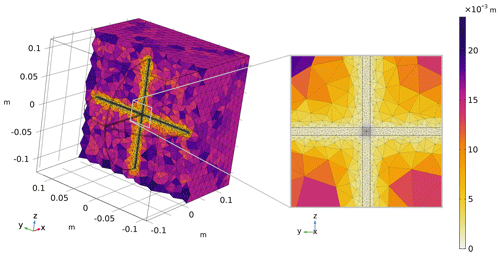Figure 2Sketch illustrating the element's size distribution for the model with connected cracks. The element's size in the crack is $\mathrm{5}×{\mathrm{10}}^{-\mathrm{5}}-\mathrm{1}×{\mathrm{10}}^{-\mathrm{3}}$ m, and in the surrounding grain material it is $\mathrm{2.4}×{\mathrm{10}}^{-\mathrm{3}}-\mathrm{1.6}×{\mathrm{10}}^{-\mathrm{2}}$ m. The element's size distribution for the model with disconnected cracks is the same.

## 4.2 Fluid pressure fields

Here and later on we deal only with a liquid-saturated pore space. The liquid has properties of glycerol (Table 1). A direct P-wave modulus test is performed to calculate dispersion and attenuation for the c33 component. (A harmonic displacement is applied to the top wall of the model in the z direction, while the normal component of the displacement is set to zero on all the other walls.) Figure 3 shows snapshots of the fluid pressure Pf in the cracks at three different frequencies, in the vertical middle slice of the model (the yz plane, red frame in Fig. 1a). For the model with connected cracks, at low frequencies, there is enough time for pressure equilibration between the cracks; thus, the pore pressure is uniform throughout the pore space (Fig. 3, LF (connected)). This is called the relaxed state. At intermediate frequencies, there is a large pressure gradient in the cracks, which corresponds to the maximum attenuation due to squirt flow between cracks (Fig. 3, Fc (connected)). At high frequencies, there is no time for fluid to move; hence, there is no fluid pressure equilibration between the vertical and horizontal cracks (Fig. 3, HF (connected)). This is called the unrelaxed state. Therefore, at high frequencies, the connected cracks behave as hydraulically isolated and the fluid highly stiffens the crack.

In the model with disconnected cracks, the fluid pressure in the cracks is the same in all three regimes, which corresponds to the unrelaxed state in the model with connected cracks. The unrelaxed state can be interpreted as the elastic limit because there is no fluid flow between the cracks, and the effective properties of the two models (connected and disconnected cracks) are the same, as will be shown in the next subsection.

## 4.3 Dispersion and attenuation

### 4.3.1 Elastic moduli

Figure 4 shows the numerical results for the complex-valued frequency-dependent components of the stiffness matrix cij(ω) (in Voigt notation) for the models with connected and disconnected cracks filled with glycerol. In the model with connected cracks, the real part of the cij component and the corresponding inverse quality factor (Eq. 6) curves show strong frequency-dependent behavior of the c22, c33 and c23 components (Fig. 4a and c). The inverse quality factor and dispersion of the c22 and c33 components coincide because the geometrical properties of the two cracks are the same (Fig. 4a) and the model is symmetric. The c11 component is nondispersive and exhibits zero attenuation. The dispersion of the c44, c55 and c66 components is negligible and these components also exhibit negligible attenuation (Fig. 4b). The c12 and c13 components are nondispersive, the c23 component exhibits strong negative dispersion and a negative inverse quality factor peak is shifted towards high frequencies compared to that of the c22 and c33 components. A similar phenomenon has been reported by in the context of two-dimensional simulations. The c23 component does not correspond to a wave mode alone; it is always used together with c22 and/or c33 components. Therefore, no wave will gain energy. This negative inverse quality factor sign for the c23 component was also verified using Kramers–Kronig relations. In other words, different components of the stiffness tensor might have positive or negative values of the ratio Im(c23)∕Re(c23) but, when we calculate the velocity and the inverse quality factor of a wave, the cumulative effect of all cij components must be physical and no negative attenuation will be observed.

Note that the width of the inverse quality factor peak (at half amplitude) for the components c22 and c33 has a 1.5 order of magnitude (Fig. 4a and 4c). It means that attenuation and dispersion due to squirt flow play a significant role over a broad frequency range even for cracks with a single aspect ratio.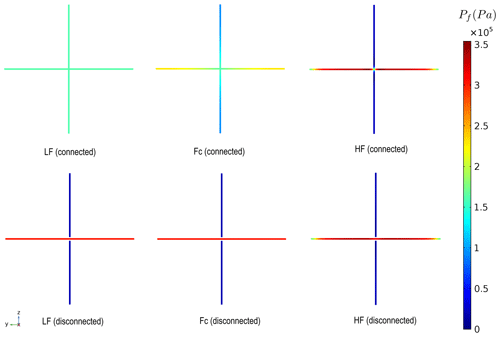Figure 3Snapshots of the fluid pressure Pf in the cracks at three different frequencies: LF – the low-frequency limit (corresponds to 101 Hz, relaxed state), Fc – intermediate frequency snapshot (corresponds to 104 Hz, close to the characteristic frequency) and HF – the high-frequency limit (corresponds to 106.5 Hz, unrelaxed state).

In the model with disconnected cracks, all components of the stiffness tensor cij(ω) (Fig. 4a and 4c) are constant across the whole frequency range and exhibit zero inverse quality factor. Furthermore, all components are approximately equal to the high-frequency values of the model with connected cracks. This is expected in the unrelaxed state because the connected cracks behave as hydraulically isolated with respect to fluid flow. A very small discrepancy between the two models at high frequencies is associated with the vertical crack partition (two thin regions of pore space replaced with stiffer grain material).

### 4.3.2 P- and S-wave velocities

Figure 5 shows the P-wave (primary wave) phase velocity as a function of the phase angle of the numerical model with connected and disconnected cracks (Fig. 1), where the zero phase angle corresponds to the vertical wave propagation (along z axis). The P- and S-wave phase velocities are calculated by solving the Christoffel equation, which represents an eigenvalue problem relating the stiffness components cij, the phase velocities of plane waves that propagate in the medium and the polarization of the waves . Considering the plane YZ, the P-wave velocity is the same for phase angles of 0 and 90; it changes with frequency only for phase angles between 0 and 90 and is maximal in the high-frequency limit at phase angle of $\mathit{\theta }=\mathrm{90}\left(±\mathrm{90}\right)$ (Fig. 5a). Furthermore, in the high-frequency limit the P-wave phase velocity coincides for the models with connected and disconnected cracks. As frequency decreases, the P-wave velocity decreases, and at 104 Hz the P-wave velocity is almost angle independent (yellow curve, Fig. 5a). It is interesting that this “local” isotropy corresponds to the maximum attenuation of the c22 and c33 components (Fig. 4). As frequency further decreases, the P-wave velocity decreases and stays nearly unchanged for the frequencies below 103.5 Hz. In the XZ plane, the P-wave phase velocity is the same for the models with connected and disconnected cracks in the high-frequency limit (Fig. 5b). For the model with connected cracks, as frequency decreases, the P-wave velocity decreases, reaching its minimum at low frequencies (101103.5 Hz).

Figures 67 show the quasi-shear (SV) and the pure shear (SH) phase velocities as functions of the phase angle of the numerical models with connected and disconnected cracks (Fig. 1). The SV wave velocity is strongly frequency dependent in both the XZ and YZ planes. The SH wave exhibits some frequency-dependent behavior in the XZ plane and is angle and frequency independent in the YZ plane. It is interesting that the SV waves in two different planes have different velocities at 0 and 90 phase angles, which is due to their different wave polarization. The SV wave in the YZ plane has the same polarization as the SH wave in the XZ plane; their velocities are equivalent at the 0 and 90 phase angles. The same conclusion is valid for the SV wave in the XZ plane and the SH wave in the YZ plane. A slight discrepancy (around 0.5 %) between the SV wave velocities for the disconnected crack model (Fig. 6, dashed red line) and the high-frequency velocity for the connected crack model (Fig. 6, green line) at phase angles of 0, 90 and 180 is due to the crack separation.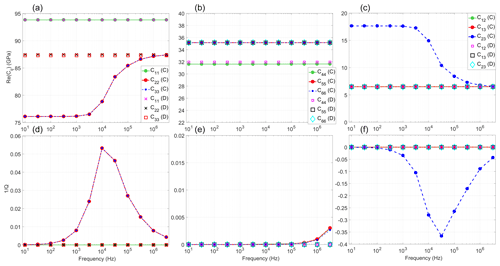Figure 4Numerical results for the connected (C) and disconnected (D) crack models: real part of the cij components versus frequency (a–c), dimensionless inverse quality factor of the cij components versus frequency (d–f). Each symbol corresponds to the test result of one numerical simulation and lines correspond to linear interpolation between discrete numerical results.

Due to the symmetry of the model, the behaviors of the P-, SV-, SH-wave phase velocities in the XZ and XY planes are identical; thus, the results in the XY plane are not shown here.

## 4.4 Quantitative analysis of the frequency-dependent anisotropy

First, we quantify the Thomsen-type anisotropic parameters (Thomsen1986) for orthorhombic media . Then, we quantify the universal elastic anisotropy index and the two parameters which define the anisotropy strength in bulk and shear modes. All these anisotropy measures highlight different frequency-dependent features of the models. Our results shown in Fig. 4 (frequency-dependent elastic moduli) are used as input to quantify these anisotropy measures.

### 4.4.1 Thomsen-type parameters

Thomsen-type anisotropic parameters (ϵ, δ, γ) describe the P-wave anisotropy – ϵ, the shape of the P-wave phase velocity at different phase angles – δ and the S-wave anisotropy – γ: each set of three parameters corresponds to one plane. Thus, for our model symmetry, there are two different planes – YZ and XZ (because the XZ plane is equivalent to the XY plane). In this study, we refer to Thomsen parameters $|\mathit{ϵ}|,|\mathit{\delta }|,|\mathit{\gamma }|\in \left[\mathrm{0},\mathrm{0.1}\right]$ as to weak elastic anisotropy ($|\cdot |$ corresponds to the absolute value), $|\mathit{ϵ}|,|\mathit{\delta }|,|\mathit{\gamma }|\in \left[\mathrm{0.1},\mathrm{0.15}\right]$ as moderate elastic anisotropy and $|\mathit{ϵ}|,|\mathit{\delta }|,|\mathit{\gamma }|\in \left[\mathrm{0.15},+\mathrm{\infty }\right]$ as strong elastic anisotropy. The choice of these intervals is based on the divergence between the exact and approximate (by using Thomsen parameters) equations for the P-wave phase velocities in cracked media.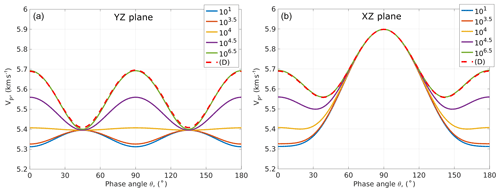Figure 5P-wave phase velocity versus phase angle in the YZ plane (a) and the XZ plane (b). Curves 101106.5 denote the frequency of the P wave for the model with connected cracks. (D) denotes the P wave for the model with disconnected cracks.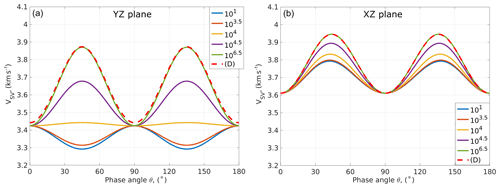Figure 6SV-wave phase velocity versus phase angle in the YZ plane (a) and the XZ plane (b). Curves 101−106.5 denote the frequency of the P wave for the model with connected cracks. (D) denotes the P wave for the model with disconnected cracks.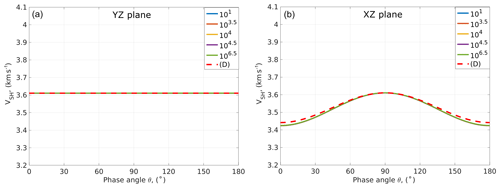Figure 7SH-wave phase velocity versus phase angle in the YZ plane (a) and the XZ plane (b). Curves 101−106.5 denote the frequency of the P wave for the model with connected cracks. (D) denotes the P wave for the model with disconnected cracks.

Figure 8 shows the Thomsen-type anisotropy parameters in the YZ and XZ planes (formulas are given in Appendix B). In the high-frequency limit, all anisotropy parameters are the same for both models with connected and disconnected cracks. Furthermore, for the model with disconnected cracks, all anisotropy parameters are frequency independent, because the stiffness tensor is frequency independent. For the model with connected cracks, several anisotropy parameters are frequency dependent due to squirt flow.

In the YZ plane, parameters ϵYZ and γYZ are zero for both models. The parameter δYZ is frequency dependent and controls the shape of the P-wave phase velocity between 0 and 90. In the high-frequency limit, δYZ exhibits the maximum negative value which corresponds to strong elastic anisotropy. As frequency decreases, δYZ also decreases reaching a zero value around 104 Hz, and then δYZ increases reaching its positive maximum at low frequencies, which corresponds to weak elastic anisotropy; the positive maximum is approximately one-third of the absolute value of its negative maximum. It is interesting that δYZ changes sign from negative to positive, which is indeed observed in the P-wave velocity behavior (Fig. 5a) as P-wave velocity changes polarity with frequency. This was also observed by in the framework of Biot's theory. This polarity change has a fully mechanical nature. In the high-frequency limit, cracks behave as hydraulically isolated and fluid highly stiffens the normal compliance of the cracks (not tangential). As frequency decreases, fluid started to flow from more compliant to stiffer cracks as a response to the applied displacement boundary condition. δ(yz)=0 corresponds to zero anisotropy; the numerator of δYZ is ${\left[{c}_{\mathrm{23}}\left(\mathit{\omega }\right)+{c}_{\mathrm{44}}\left(\mathit{\omega }\right)\right]}^{\mathrm{2}}-{\left[{c}_{\mathrm{33}}\left(\mathit{\omega }\right)-{c}_{\mathrm{44}}\left(\mathit{\omega }\right)\right]}^{\mathrm{2}}$ (see Appendix B). Therefore, for zero anisotropy, c23(ω)+c44(ω) must be equal to c33(ω)−c44(ω). The function c44(ω) is constant across the whole frequency range, c23(ω) is strictly decreasing with frequency and c33(ω) is strictly increasing with frequency (Fig. 4). At a certain frequency (here it is at ∼104 Hz), the c33 and c23 components are in such a combination that ${c}_{\mathrm{23}}\left({\mathrm{10}}^{\mathrm{4}}\right)+{c}_{\mathrm{44}}\left({\mathrm{10}}^{\mathrm{4}}\right)\approx {c}_{\mathrm{33}}\left({\mathrm{10}}^{\mathrm{4}}\right)-{c}_{\mathrm{44}}\left({\mathrm{10}}^{\mathrm{4}}\right)$, so δYZ=0, and the P-wave velocity in the YZ plane behaves as in a fully isotropic media.

In the XZ plane, ϵXZ and δXZ are frequency dependent in the model with connected cracks. ϵXZ exhibits moderate elastic anisotropy at low frequencies, while δXZ exhibits moderate elastic anisotropy at high frequencies. Other parameters are frequency independent and exhibit certain nonzero values from weak to moderate elastic anisotropy.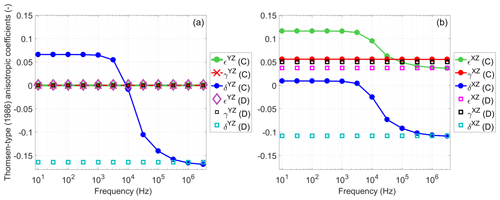Figure 8Thomsen-type anisotropic parameters in the YZ (a) and XZ (b) planes.

### 4.4.2 The universal elastic anisotropy index

The universal elastic anisotropy index AU is widely used to measure the anisotropy strength in crystallography, engineering and materials science. This parameter is designed to evaluate the anisotropy strength of crystals having any elastic symmetry class . Since AU is a scalar, it gives a simple and fast identification of the overall anisotropy strength of a model. AU=0 corresponds to zero anisotropy of a model, while the discrepancy of AU from zero defines the anisotropy strength and accounts for both the shear and the bulk contributions simultaneously. In analogy to the universal elastic anisotropy index, two other parameters are adopted which define the anisotropy strength in bulk Abulk and in shear Ashear. As far as we are concerned, these parameters have not been widely used in earth sciences: only a few studies were found. applied the universal elastic anisotropy index and two similar parameters for bulk and shear to study seismic properties and anisotropy of the continental crust. , and applied AU to quantify the elastic anisotropy of polycrystals. A brief review of these anisotropic measures and all necessary equations for their calculation are provided in Appendix C.

Figure 9 shows the universal elastic anisotropy index AU and the anisotropy measures in bulk Abulk(ω) and shear Ashear. For the model with disconnected cracks, AU is constant and frequency independent (Fig. 9, black line). Because AU has a certain small value (about 0.058), the model with disconnected cracks exhibits a certain small anisotropy. For the model with connected cracks, AU in the high-frequency limit is almost the same as for the model with disconnected cracks (Fig. 9, red line). (The nature of the discrepancy is related to the region containing the crack intersection.) For the model with connected cracks, the overall anisotropy slightly decreases towards lower frequencies until 104.3 Hz, reaching its minimum of 0.048 (Fig. 9, red line). This local minimum indeed corresponds to the c23 attenuation peak (Fig. 4c). Then, still towards lower frequencies, AU(ω) increases reaching its maximum of 0.083 at frequencies below 103 Hz (Fig. 9, red line). Thus, the overall anisotropy of the model mainly increases due to squirt flow between the cracks, so the crack connectivity increases the overall anisotropy of the model towards low frequencies.

The anisotropy measure in bulk Abulk is constant and frequency independent for the models with connected and disconnected cracks (Fig. 9b). It means that fluid flow does not affect bulk properties of the model or the anisotropy strength in bulk. On the other hand, the anisotropy measure in shear Ashear(ω) basically reproduces the behavior of the universal elastic anisotropy index measure AU. Therefore, one can conclude that the fluid flow changes anisotropy in shear mode but not in bulk mode.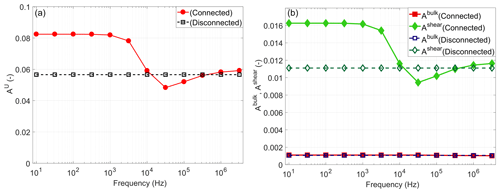Figure 9The universal elastic anisotropy index measure AU versus frequency (a) and the anisotropy measures in bulk and shear (Abulk and Ashear(b). These plots show that the overall anisotropy of the model increases in the low frequencies due to fluid flow.

5 Discussion

## 5.1 Elastic anisotropy

Thomsen-type anisotropic parameters provide a very detailed description of the velocity anisotropy in different planes. Most importantly, only a limited number of the stiffness tensor coefficients are needed to calculate ϵ, δ and γ in each plane. Thus, Thomsen parameters can be used to quantify the medium anisotropy using seismic data. On the other hand, when all components of the stiffness tensor are known and the model's symmetry is low, it is difficult to analyze the overall anisotropy due to a large number of Thomsen parameters. For example, if the model exhibits orthorhombic symmetry, one should analyze nine Thomsen anisotropic parameters (three in each plane). Due to a large number of Thomsen parameters in this study, it is difficult to evaluate whether the overall medium's anisotropy is increasing or decreasing with frequency and how far the current model is from the closest isotropy. Thus, in addition to (or instead of) the Thomsen-type anisotropic parameters, the universal elastic anisotropy index can be used. The universal elastic anisotropy index AU and the related measures in bulk, Abulk, and shear, Ashear, provide the overall description of the anisotropy strength regardless of the model's complexity. The calculation of these parameters is as simple as the calculation of the Thomsen parameters. An obvious disadvantage of the universal elastic anisotropy index (and related measures) is that it requires knowledge of the full stiffness tensor. Thus, this anisotropic measure can be useful to evaluate results of numerical simulations of laboratory experiments and for measuring the anisotropy of single crystals.

The analysis of two sets of anisotropic measures shows that (i) the overall anisotropy of the model with connected cracks (Fig. 1) mainly increases due to squirt flow towards low frequencies with a slight local decrease at intermediate frequencies (Fig. 9a); (ii) in the YZ plane, the magnitude of the “delta” anisotropy parameter decreases, reaches zero, and then increases again (reaching approximately one-third of its high-frequency value) towards low frequencies (Fig. 8a, blue curve); and (iii) in the XZ plane, the “delta” anisotropy parameter decreases towards low frequencies (Fig. 8b, blue curve), while the “epsilon” anisotropy parameter increases (Fig. 8b, green curve).

## 5.2 Comparison with previous works

In this study, we numerically solve a coupled fluid–solid deformation problem at the pore scale. If we consider the mesoscopic scale scenario and use Biot's (1941) equations, the fluid flow effects on the effective moduli are equivalent to that of the coupled elastic Stokes equations (as in the present study), as it was shown by . The frequency-dependent anisotropy due to fluid flow at the mesoscopic scale for orthogonal fracture sets with different degrees of connectivity was numerically studied by , but their study was limited to two dimensions. The main conclusion of is that the anisotropy decreases with fracture connectivity in the seismic frequency band due to fluid flow between connected fractures. The YZ plane in the present 3D numerical model is reasonably equivalent to the 2D numerical model of as well as the physical mechanism under consideration. The results of are reflected in Fig. 5 of this study. However, a more in-depth analysis shows that the anisotropy in the YZ plane decreases, reaches zero and then increases again towards low frequencies due to squirt flow (Fig. 5a, green, yellow and blue curves, respectively). Moreover, our present study shows that the overall anisotropy of the model with cracks of finite length actually increases due to fluid flow between interconnected cracks (Figs. 8, 9). This conclusion is not universal and is valid only for a specific set of model parameters.

performed a more detailed study of seismic anisotropy for a similar fracture geometry in two dimensions, as in the study of , specifying that the decrease in anisotropy is described by the anisotropy parameter δ while ϵ is zero. Furthermore, they observed a polarity change of the P-wave phase velocity behavior with frequency. In the present study, the “delta” anisotropy parameter in the YZ plane is more pronounced in the low-frequency limit (Fig. 5a, blue curve) compared to the work of due to different material properties and the three-dimensional nature of the present model configuration.

In summary, fluid flow effects on seismic anisotropy are nonlinear with a possible increase or decrease in the elastic anisotropy at different frequencies. These two extreme cases, the maximum negative and the maximum positive δ parameter (and hence P-wave velocity) in the YZ plane, correspond to the relaxed and unrelaxed states. In other words, seismic anisotropy may behave completely different in different scenarios; therefore, more studies should be performed, especially with the sensitivity analysis of model parameters.

## 5.3 A qualitative comparison against analytical models

Numerical simulations are useful but analytical models are especially attractive since they help us to better understand the physics and do not require sophisticated numerical simulations. The limitations of the analytical solutions are restricted to simple pore space geometry, and some assumptions related to physics are needed to derive the closed form analytical formulas. Such a comparison of the numerical results against an analytical solution has been performed by for a different pore space geometry. Unfortunately, there is no analytical solution for the present study considering a periodic distribution of intersecting cracks in three dimensions. But the qualitative comparison of the low- and high-frequency limits (which correspond to relaxed and unrelaxed states) is possible . Several analytical studies show that the anisotropy (described by Thompson's parameters) is, in general, more pronounced at high frequencies than at low frequencies . In the relaxed state, one can calculate the effective dry elastic moduli and use Gassmann's equations to obtain the effective moduli of the saturated medium. In the unrelaxed state, one can calculate the effective elastic moduli by restricting fluid flow (by using zero displacement boundary conditions in the crack intersections). The low- and high-frequency limits for elastic moduli have been calculated using these semi-analytical approaches, and numerical results have been reproduced.

6 Conclusions

We have numerically calculated the frequency-dependent elastic moduli of a fluid-saturated porous medium caused by squirt flow. The considered 3D numerical models consist of two perpendicular connected or disconnected cracks embedded in a solid grain material. Cracks are represented by very flat cylinders filled with a fluid. Grains are described as a linear isotropic elastic material, while the fluid phase is described by the quasistatic linearized compressible Navier–Stokes momentum equation.

We showed that seismic velocities are azimuth, angle and frequency dependent due to squirt flow between connected cracks. The resulting elastic frequency-dependent anisotropy was analyzed by using the Thomsen-type anisotropic parameters and the universal elastic anisotropy index. The latter is a scalar parameter which can be used to analyze the overall anisotropy of the model and its divergence from the closest isotropy. We showed that the seismic anisotropy may locally decrease as well as increase due to squirt flow in one specific plane. However, the overall anisotropy of the model mainly increases due to squirt flow between the cracks towards low frequencies. In the model with disconnected cracks, no fluid flow occurs, and thus the effective properties of the model correspond to the elastic limit. The elastic limit is equivalent to the high-frequency limit for the model with connected cracks. Seismic velocities are only azimuth and angle dependent as for a fully elastic material, and they are independent of frequency. In summary, squirt flow does affect effective mechanical properties of cracked rocks and thus seismic velocity anisotropy. Given that seismic anisotropy variations with frequency are very sensitive to the pore space geometry and material properties, it is difficult to make a general prediction. According to our study, the effective frequency-dependent response of a cracked medium is different in different planes. The local response (in a certain plane) is controlled by crack orientation, which is the key parameter. The magnitude of the frequency-dependent response (i.e., the dispersion and attenuation) is controlled by crack compliances, crack porosity and their fluid content. (Dry or liquid-saturation conditions will cause completely different behavior.) Most importantly, crack porosity is a very important parameter in fluid-saturated rocks (contrary to dry rocks) since it defines the volume of fluid which may flow due to wave propagation, causing wave attenuation and dispersion.

Appendix A: Boundary conditions for cij off-diagonal components

Let us consider a cuboid, volume $V=\left(\mathrm{0},Lx\right)×\left(\mathrm{0},Ly\right)×\left(\mathrm{0},Lz\right)$ and Γ its boundary, $\mathrm{\Gamma }={\mathrm{\Gamma }}^{xz\mathrm{0}}\cup {\mathrm{\Gamma }}^{xzL}\cup {\mathrm{\Gamma }}^{yz\mathrm{0}}\cup {\mathrm{\Gamma }}^{yzL}\cup {\mathrm{\Gamma }}^{xy\mathrm{0}}\cup {\mathrm{\Gamma }}^{xyL}$, where Γxz0 represents an xz with zero coordinate, ΓxzL represents an xz with Ly coordinate, etc. There are six planes in total.

The mixed test for the c13 component can be derived from the anisotropic stress–strain relation (Hooke's law) (similarly to the c13 component in a VTI medium; ); ΓxyL is set to uzzu; uxx and uyy are free;
ΓyzL is set to uxxu; and uzz and uyy are free,
where $\mathrm{\Delta }u={\mathrm{10}}^{-\mathrm{6}}$. In the other four planes, the normal component of the displacement is set to zero and other components are free. The the stress–strain relation for the σ1 stress component is

$\begin{array}{}\text{(A1)}& 〈{\mathit{\sigma }}_{\mathrm{1}}〉={c}_{\mathrm{11}}〈{\mathit{ϵ}}_{\mathrm{1}}〉+{c}_{\mathrm{12}}〈{\mathit{ϵ}}_{\mathrm{2}}〉+{c}_{\mathrm{13}}〈{\mathit{ϵ}}_{\mathrm{3}}〉.\end{array}$

Using the abovementioned boundary conditions and setting ϵ2〉=0, Eq. (A1) becomes

$\begin{array}{}\text{(A2)}& 〈{\mathit{\sigma }}_{\mathrm{1}}〉={c}_{\mathrm{11}}〈{\mathit{ϵ}}_{\mathrm{1}}〉+{c}_{\mathrm{13}}〈{\mathit{ϵ}}_{\mathrm{3}}〉.\end{array}$

Equation (A2) can be solved for the c13 component; the solution is

$\begin{array}{}\text{(A3)}& {c}_{\mathrm{13}}=\frac{〈{\mathit{\sigma }}_{\mathrm{1}}〉}{〈{\mathit{ϵ}}_{\mathrm{1}}〉}-{c}_{\mathrm{11}}.\end{array}$

Equation (A3) is used to calculate the c13 component (c11 is taken from the direct tests).

The mixed test for the c23 (in this numerical model, the c23 is dispersive) component again can be derived from the anisotropic stress–strain relation (Hooke's law) (similarly to the previous test). ΓxyL is set to uzzu; uxx,uyy are free;
ΓxzL is set to uyyu; and uzz and uxx are free.

In the other four planes, the normal component of the displacement is set to zero, other components are free. Then, using the following equation

$\begin{array}{}\text{(A4)}& {c}_{\mathrm{23}}=\frac{〈{\mathit{\sigma }}_{\mathrm{2}}〉}{〈{\mathit{ϵ}}_{\mathrm{2}}〉}-{c}_{\mathrm{22}},\end{array}$

the c23 component is calculated (c22 is taken from the direct test). Equations (A3)–(A4) are found from Hooke's law considering nonzero strains in the x and z (in y and z) directions and then solving a system of two equations analytically.

Appendix B: Thomsen-type anisotropic parameters

Thomsen-type anisotropic parameters (Thomsen1986) are widely used in the applied geophysics community. Thomsen weak anisotropy parameters were originally developed for vertical transverse isotropic media (Thomsen1986). A natural extension of these parameters to orthorhombic media was suggested by and . These parameters correspond to the anisotropy of the compression and shear waves in orthorhombic media in different Cartesian propagation planes. In the YZ plane, Thomsen-type anisotropic parameters are

$\begin{array}{}\text{(B1)}& \begin{array}{rl}{\mathit{ϵ}}^{\left(YZ\right)}\left(\mathit{\omega }\right)=& \frac{{c}_{\mathrm{22}}\left(\mathit{\omega }\right)-{c}_{\mathrm{33}}\left(\mathit{\omega }\right)}{\mathrm{2}{c}_{\mathrm{33}}\left(\mathit{\omega }\right)},\phantom{\rule{0.25em}{0ex}}\phantom{\rule{0.25em}{0ex}}{\mathit{\gamma }}^{\left(YZ\right)}\left(\mathit{\omega }\right)\\ =& \frac{{c}_{\mathrm{66}}\left(\mathit{\omega }\right)-{c}_{\mathrm{55}}\left(\mathit{\omega }\right)}{\mathrm{2}{c}_{\mathrm{55}}\left(\mathit{\omega }\right)},\end{array}\end{array}$

and

$\begin{array}{}\text{(B2)}& {\mathit{\delta }}^{\left(YZ\right)}\left(\mathit{\omega }\right)=\frac{{\left[{c}_{\mathrm{23}}\left(\mathit{\omega }\right)+{c}_{\mathrm{44}}\left(\mathit{\omega }\right)\right]}^{\mathrm{2}}-{\left[{c}_{\mathrm{33}}\left(\mathit{\omega }\right)-{c}_{\mathrm{44}}\left(\mathit{\omega }\right)\right]}^{\mathrm{2}}}{\mathrm{2}{c}_{\mathrm{33}}\left(\mathit{\omega }\right)\left[{c}_{\mathrm{33}}\left(\mathit{\omega }\right)-{c}_{\mathrm{44}}\left(\mathit{\omega }\right)\right]}.\end{array}$

In the XZ plane, Thomsen-type anisotropic parameters are

$\begin{array}{}\text{(B3)}& \begin{array}{rl}{\mathit{ϵ}}^{\left(XZ\right)}\left(\mathit{\omega }\right)=& \frac{{c}_{\mathrm{11}}\left(\mathit{\omega }\right)-{c}_{\mathrm{33}}\left(\mathit{\omega }\right)}{\mathrm{2}{c}_{\mathrm{33}}\left(\mathit{\omega }\right)},\phantom{\rule{0.25em}{0ex}}\phantom{\rule{0.25em}{0ex}}{\mathit{\gamma }}^{\left(XZ\right)}\left(\mathit{\omega }\right)\\ =& \frac{{c}_{\mathrm{66}}\left(\mathit{\omega }\right)-{c}_{\mathrm{44}}\left(\mathit{\omega }\right)}{\mathrm{2}{c}_{\mathrm{44}}\left(\mathit{\omega }\right)},\end{array}\end{array}$

and

$\begin{array}{}\text{(B4)}& {\mathit{\delta }}^{\left(XZ\right)}\left(\mathit{\omega }\right)=\frac{{\left[{c}_{\mathrm{13}}\left(\mathit{\omega }\right)+{c}_{\mathrm{55}}\left(\mathit{\omega }\right)\right]}^{\mathrm{2}}-{\left[{c}_{\mathrm{33}}\left(\mathit{\omega }\right)-{c}_{\mathrm{55}}\left(\mathit{\omega }\right)\right]}^{\mathrm{2}}}{\mathrm{2}{c}_{\mathrm{33}}\left(\mathit{\omega }\right)\left[{c}_{\mathrm{33}}\left(\mathit{\omega }\right)-{c}_{\mathrm{55}}\left(\mathit{\omega }\right)\right]}.\end{array}$
Appendix C: The universal elastic anisotropy index parameter

Assuming that one deals with an anisotropic frequency-dependent effective fourth-rank stiffness tensor C (might be frequency dependent CC(ω)), a compliance tensor is defined as $\mathbf{S}\left(\mathit{\omega }\right)=\mathbf{C}\left(\mathit{\omega }{\right)}^{-\mathrm{1}}$. Then, for each frequency, the effective single orientation fourth-rank stiffness and compliance tensors are uniformly distributed and the isotropic stiffness and compliance tensors are calculated. Averaging the single orientation stiffness tensor belongs to the Voigt assumption, which is the theoretical maximum value of the isotropic elastic moduli. On the other hand, averaging the single orientation compliance tensor belongs to the Reuss assumption which is the theoretical minimum value of the isotropic elastic moduli. The resulting isotropic tensors can be expressed in terms of the spherical and deviatoric parts corresponding to bulk K and shear moduli μ:

$\begin{array}{}\text{(C1)}& {\mathbf{C}}^{\mathrm{V}}\left(\mathit{\omega }\right)=\mathrm{3}{K}^{\mathrm{V}}\mathbf{J}+\mathrm{2}{\mathit{\mu }}^{\mathrm{V}}\mathbf{D}\end{array}$

and

$\begin{array}{}\text{(C2)}& {\mathbf{S}}^{\mathrm{R}}\left(\mathit{\omega }\right)=\frac{\mathrm{1}}{\mathrm{3}{K}^{\mathrm{R}}}\mathbf{J}+\frac{\mathrm{1}}{\mathrm{2}{\mathit{\mu }}^{\mathrm{R}}}\mathbf{D},\end{array}$

where the superscripts “V” and “R” correspond to Voigt and Reuss estimates, respectively. J and D are the spherical (volumetric) and deviatoric parts of the symmetric unit fourth-order tensor.

The double contraction of the scalar product (quadruple contraction) of Eqs. (C1) and (C2) gives

$\begin{array}{}\text{(C3)}& {\mathbf{C}}^{\mathrm{V}}\left(\mathit{\omega }\right)::{\mathbf{S}}^{\mathrm{R}}\left(\mathit{\omega }\right)=\frac{{K}^{\mathrm{V}}\left(\mathit{\omega }\right)}{{K}^{\mathrm{R}}\left(\mathit{\omega }\right)}+\mathrm{5}\frac{{\mathit{\mu }}^{\mathrm{V}}\left(\mathit{\omega }\right)}{{\mathit{\mu }}^{\mathrm{R}}\left(\mathit{\omega }\right)}.\end{array}$

If the effective stiffness tensor is isotropic, then ${\mathbf{C}}^{\mathrm{V}}\left(\mathit{\omega }\right)={\left({\mathbf{S}}^{\mathrm{R}}\left(\mathit{\omega }\right)\right)}^{-\mathrm{1}}$ and ${K}^{\mathrm{V}}/{K}^{\mathrm{R}}={\mathit{\mu }}^{\mathrm{V}}/{\mathit{\mu }}^{\mathrm{R}}=\mathrm{1}$. Therefore, when the effective stiffness tensor is isotropic, the value of Eq. C3 equals to 6 and this value increases when the effective stiffness tensor becomes anisotropic. Thus, the universal elastic anisotropy index measure AU is defined as :

$\begin{array}{}\text{(C4)}& {A}^{\mathrm{U}}\left(\mathit{\omega }\right)=\frac{{K}^{\mathrm{V}}\left(\mathit{\omega }\right)}{{K}^{\mathrm{R}}\left(\mathit{\omega }\right)}+\mathrm{5}\frac{{\mathit{\mu }}^{\mathrm{V}}\left(\mathit{\omega }\right)}{{\mathit{\mu }}^{\mathrm{R}}\left(\mathit{\omega }\right)}-\mathrm{6}\ge \mathrm{0},\end{array}$

In geophysics, the separation of the elastic anisotropy measure in bulk and shear modes is necessary because rocks might exhibit different frequency dependencies due to bulk and shear deformations. Therefore, in analogy to the universal elastic anisotropy index measure AU, the anisotropy measures in bulk Abulk(ω) and shear Ashear(ω) can be defined as

$\begin{array}{}\text{(C5)}& {A}^{\text{bulk}}\left(\mathit{\omega }\right)=\frac{{K}^{\mathrm{V}}\left(\mathit{\omega }\right)}{{K}^{\mathrm{R}}\left(\mathit{\omega }\right)}-\mathrm{1}\end{array}$

and

$\begin{array}{}\text{(C6)}& {A}^{\text{shear}}\left(\mathit{\omega }\right)=\frac{{G}^{\mathrm{V}}\left(\mathit{\omega }\right)}{{G}^{\mathrm{R}}\left(\mathit{\omega }\right)}-\mathrm{1}.\end{array}$

These two parameters, Abulk(ω) and Ashear(ω), obey the same interpretation as the universal elastic anisotropy index measure: Abulk=0 (Ashear=0) corresponds to zero bulk (shear) anisotropy of the model, while the discrepancy of Abulk (Ashear) from zero anisotropy defines the anisotropy strength in bulk (shear). The Voigt and Reuss estimates (KV, KR, μV and μR) can be calculated via simple algebraic formulas for different symmetry classes which can be found elsewhere, e.g., in for orthorhombic symmetry, in for tetragonal symmetry and in for cubic symmetry. Thus, for orthorhombic symmetry (lowest possible symmetry created by two perpendicular sets of cracks embedded into an isotropic material), the Voigt and Reuss bulk moduli are (written for the components of stiffness (compliance) matrices cij(sij), in Voigt notation)

$\begin{array}{}\text{(C7)}& {K}^{\mathrm{V}}=\frac{\mathrm{1}}{\mathrm{9}}\left[\left({c}_{\mathrm{11}}+{c}_{\mathrm{22}}+{c}_{\mathrm{33}}\right)+\mathrm{2}\left({c}_{\mathrm{12}}+{c}_{\mathrm{13}}+{c}_{\mathrm{23}}\right)\right]\end{array}$

and

$\begin{array}{}\text{(C8)}& {K}^{\mathrm{R}}={\left[\left({s}_{\mathrm{11}}+{s}_{\mathrm{22}}+{s}_{\mathrm{33}}\right)+\mathrm{2}\left({s}_{\mathrm{12}}+{s}_{\mathrm{13}}+{s}_{\mathrm{23}}\right)\right]}^{-\mathrm{1}}.\end{array}$

Similarly, the Voigt and Reuss shear moduli are (in Voigt notation)

$\begin{array}{}\text{(C9)}& \begin{array}{rl}{\mathit{\mu }}^{\mathrm{V}}=& \frac{\mathrm{1}}{\mathrm{15}}\left[\left({c}_{\mathrm{11}}+{c}_{\mathrm{22}}+{c}_{\mathrm{33}}-{c}_{\mathrm{12}}-{c}_{\mathrm{13}}-{c}_{\mathrm{23}}\right)\right\\ +& \mathrm{3}\left({c}_{\mathrm{44}}+{c}_{\mathrm{55}}+{c}_{\mathrm{66}}\right)]\end{array}\end{array}$

and

$\begin{array}{}\text{(C10)}& \begin{array}{rl}{\mathit{\mu }}^{\mathrm{R}}=& \mathrm{15}\left[\mathrm{4}\left({s}_{\mathrm{11}}+{s}_{\mathrm{22}}+{s}_{\mathrm{33}}-{s}_{\mathrm{12}}-{s}_{\mathrm{13}}-{s}_{\mathrm{23}}\right)\right\\ +& \mathrm{3}\left({s}_{\mathrm{44}}+{s}_{\mathrm{55}}+{s}_{\mathrm{66}}\right){}^{-\mathrm{1}}.\end{array}\end{array}$

Equations (C7)–(C10) are valid for orthorhombic symmetry and for higher symmetries: tetragonal, transverse isotropy and cubic. Thus, for evaluating the universal elastic anisotropy index AU and the anisotropy measures in bulk Abulk(ω) and shear Ashear(ω), one can use Eqs. (C7)–(C10) to calculate the Voigt and Reuss estimates (KV, KR, μV and μR) and then calculate AU using Eq. (C4) and Abulk(ω) and Ashear(ω) using Eqs. (C5) and (C6), respectively.

Code and data availability
Code and data availability.

This is a theoretical study. Data are available from the authors upon reasonable request.

Author contributions
Author contributions.

YA performed the numerical simulations and wrote the article. The idea of this project was first inspired by the paper by . A detailed project was planned by YA and BQ. EC, SL and BQ provided many ideas and suggestions which influenced the project path and helped writing the article.

Competing interests
Competing interests.

The authors declare that they have no conflict of interest.

Acknowledgements
Acknowledgements.

This research is funded by the Swiss National Science Foundation, project number 172691. Yury Alkhimenkov thanks Germán Rubino (CONICET, Centro Atómico Bariloche, Argentina) for fruitful discussions on the frequency-dependent anisotropy due to fluid flow, Nicolás D. Barbosa (University of Geneva, Switzerland) for fruitful discussions regarding the polarity change of the P-wave velocity with frequency and Irina Bayuk (Russian Academy of Sciences, Russia) for enlightening discussions regarding the fourth-rank tensor averaging and the elastic symmetry classes.

Financial support
Financial support.

This research has been supported by the Swiss National Science Foundation (grant no. 172691).

Review statement
Review statement.

This paper was edited by Susanne Buiter and reviewed by Yves Gueguen and Vishal Das.

References

Adelinet, M., Fortin, J., Guéguen, Y., Schubnel, A., and Geoffroy, L.: Frequency and fluid effects on elastic properties of basalt: Experimental investigations, Geophys. Res. Lett., 37, L02303, https://doi.org/10.1029/2009GL041660, 2010. a

Alkhimenkov, Y., Caspari, E., Gurevich, B., Barbosa, N. D., Glubokovskikh, S., Hunziker, J., and Quintal, B.: Frequency-dependent attenuation and dispersion caused by squirt flow: Three-dimensional numerical study, Geophysics, 85, 1–71, 2020. a, b, c, d, e, f

Almqvist, B. S. and Mainprice, D.: Seismic properties and anisotropy of the continental crust: predictions based on mineral texture and rock microstructure, Rev. Geophys., 55, 367–433, 2017. a

Amalokwu, K., Best, A. I., and Chapman, M.: Effects of aligned fractures on the response of velocity and attenuation ratios to water saturation variation: a laboratory study using synthetic sandstones, Geophys. Pros., 64, 942–957, 2016. a

Andrä, H., Combaret, N., Dvorkin, J., Glatt, E., Han, J., Kabel, M., Keehm, Y., Krzikalla, F., Lee, M., Madonna, C., et al.: Digital rock physics benchmarks – Part I: Imaging and segmentation, Comput. Geosci., 50, 25–32, 2013a. a

Andrä, H., Combaret, N., Dvorkin, J., Glatt, E., Han, J., Kabel, M., Keehm, Y., Krzikalla, F., Lee, M., Madonna, C., et al.: Digital rock physics benchmarks – Part II: Computing effective properties, Comput. Geosci., 50, 33–43, 2013b. a

Bakulin, A., Grechka, V., and Tsvankin, I.: Estimation of fracture parameters from reflection seismic data – Part II: Fractured models with orthorhombic symmetry, Geophysics, 65, 1803–1817, 2000a. a, b

Bakulin, A., Grechka, V., and Tsvankin, I.: Estimation of fracture parameters from reflection seismic data – Part II: Fractured models with orthorhombic symmetry, Geophysics, 65, 1803–1817, 2000b. a

Barbosa, N. D., Rubino, J. G., Caspari, E., and Holliger, K.: Sensitivity of seismic attenuation and phase velocity to intrinsic background anisotropy in fractured porous rocks: A numerical study, J. Geophys. Res.-Sol. Ea., 122, 8181–8199, 2017. a, b, c, d, e

Best, A. I., Sothcott, J., and McCann, C.: A laboratory study of seismic velocity and attenuation anisotropy in near-surface sedimentary rocks, Geophys. Pros., 55, 609–625, 2007. a

Biot, M. A.: Generalized theory of acoustic propagation in porous dissipative media, J. Acoust. Soc. Am., 34, 1254–1264, 1962. a

Brajanovski, M., Gurevich, B., and Schoenberg, M.: A model for P-wave attenuation and dispersion in a porous medium permeated by aligned fractures, Geophys. J. Int., 163, 372–384, 2005. a

Carcione, J. M., Gurevich, B., Santos, J. E., and Picotti, S.: Angular and frequency-dependent wave velocity and attenuation in fractured porous media, Pure Appl. Geophys., 170, 1673–1683, 2013. a

Caspari, E., Novikov, M., Lisitsa, V., Barbosa, N. D., Quintal, B., Rubino, J. G., and Holliger, K.: Attenuation mechanisms in fractured fluid-saturated porous rocks: a numerical modelling study, Geophys. Pros., 67, 935–955, 2019. a

Chapman, M.: Frequency-dependent anisotropy due to meso-scale fractures in the presence of equant porosity, Geophys. Pros., 51, 369–379, 2003. a

Chapman, M., Zatsepin, S. V., and Crampin, S.: Derivation of a microstructural poroelastic model, Geophys. J. Int., 151, 427–451, 2002. a

Chapman, S., Borgomano, J. V., Yin, H., Fortin, J., and Quintal, B.: Forced oscillation measurements of seismic wave attenuation and stiffness moduli dispersion in glycerine-saturated Berea sandstone, Geophys. Pros., 67, 956–968, 2019. a

Das, V., Mukerji, T., and Mavko, G.: Numerical simulation of coupled fluid-solid interaction at the pore scale: A digital rock-physics technology, Geophysics, 84, 71–81, 2019. a, b

Duffy, T. S.: Single-crystal elastic properties of minerals and related materials with cubic symmetry, American Mineralogist: Journal of Earth and Planetary Materials, 103, 977–988, 2018. a, b

Dvorkin, J., Mavko, G., and Nur, A.: Squirt flow in fully saturated rocks, Geophysics, 60, 97–107, 1995. a, b

Fedorov, F. I.: Theory of elastic waves in crystals, 1968. a

Feng, J., Xiao, B., Zhou, R., Pan, W., and Clarke, D. R.: Anisotropic elastic and thermal properties of the double perovskite slab–rock salt layer Ln2SrAl2O7 (Ln=La, Nd, Sm, Eu, Gd or Dy) natural superlattice structure, Acta Material., 60, 3380–3392, 2012. a

Grab, M., Quintal, B., Caspari, E., Maurer, H., and Greenhalgh, S.: Numerical modeling of fluid effects on seismic properties of fractured magmatic geothermal reservoirs, Solid Earth, 8, 255–279, https://doi.org/10.5194/se-8-255-2017, 2017. a

Grechka, V. and Kachanov, M.: Effective elasticity of rocks with closely spaced and intersecting cracks, Geophysics, 71, 85–91, 2006. a, b

Guéguen, Y. and Sarout, J.: Crack-induced anisotropy in crustal rocks: predicted dry and fluid-saturated Thomsen's parameters, Phys. Earth Planet. In., 172, 116–124, 2009. a, b

Guéguen, Y. and Sarout, J.: Characteristics of anisotropy and dispersion in cracked medium, Tectonophysics, 503, 165–172, 2011. a, b

Guo, J., Rubino, J. G., Glubokovskikh, S., and Gurevich, B.: Effects of fracture intersections on seismic dispersion: theoretical predictions versus numerical simulations, Geophys. Pros., 65, 1264–1276, 2017. a, b

Guo, J., Rubino, J. G., Glubokovskikh, S., and Gurevich, B.: Dynamic seismic signatures of saturated porous rocks containing two orthogonal sets of fractures: theory versus numerical simulations, Geophys. J. Int., 213, 1244–1262, 2018. a

Gurevich, B., Makarynska, D., de Paula, O. B., and Pervukhina, M.: A simple model for squirt-flow dispersion and attenuation in fluid-saturated granular rocks, Geophysics, 75, 109–120, 2010. a, b

Hunziker, J., Favino, M., Caspari, E., Quintal, B., Rubino, J. G., Krause, R., and Holliger, K.: Seismic attenuation and stiffness modulus dispersion in porous rocks containing stochastic fracture networks, J. Geophys. Res.-Sol. Ea., 123, 125–143, 2018. a

Kachanov, M. and Sevostianov, I.: Micromechanics of materials, with applications, Vol. 249, Springer, 712 pp. https://doi.org/10.1007/978-3-319-76204-3, 2018. a

Kube, C. M. and De Jong, M.: Elastic constants of polycrystals with generally anisotropic crystals, J. Appl. Phys., 120, 165105, https://doi.org/10.1063/1.4965867, 2016. a

Landau, L. D. and Lifshitz, E.: Course of theoretical physics, Vol. 6, Fluid Mechanics, London, 554 pp., https://doi.org/10.1016/C2013-0-03799-1, 1959a. a

Landau, L. D. and Lifshitz, E. M.: Course of Theoretical Physics Vol. 7, Theory and Elasticity, Pergamon press, 187 pp., 1959b. a

Lissa, S., Barbosa, N. D., Rubino, J. G., and Quintal, B.: Seismic attenuation and dispersion in poroelastic media with fractures of variable aperture distributions, Solid Earth, 10, 1321–1336, https://doi.org/10.5194/se-10-1321-2019, 2019. a

Markov, A., Abaimov, S., Sevostianov, I., Kachanov, M., Kanaun, S., and Akhatov, I.: The effect of multiple contacts between crack faces on crack contribution to the effective elastic properties, Int. J. Solids Struct., 163, 75–86, 2019. a

Masson, Y. J. and Pride, S.: On the correlation between material structure and seismic attenuation anisotropy in porous media, J. Geophys. Res.-Sol. Ea., 119, 2848–2870, 2014. a

Maultzsch, S., Chapman, M., Liu, E., and Li, X. Y.: Modelling frequency-dependent seismic anisotropy in fluid-saturated rock with aligned fractures: implication of fracture size estimation from anisotropic measurements, Geophys. Pros., 51, 381–392, 2003. a

Mavko, G. and Jizba, D.: Estimating grain-scale fluid effects on velocity dispersion in rocks, Geophysics, 56, 1940–1949, 1991. a

Mavko, G. and Nur, A.: Melt squirt in the asthenosphere, J. Geophys. Res., 80, 1444–1448, 1975. a

Mavko, G., Mukerji, T., and Dvorkin, J.: The rock physics handbook: Tools for seismic analysis of porous media, Cambridge university press, https://doi.org/10.1017/CBO9780511626753, 511 pp., 2009. a

Mayr, S. I. and Burkhardt, H.: Ultrasonic properties of sedimentary rocks: effect of pressure, saturation, frequency and microcracks, Geophys. J. Int., 164, 246–258, 2006. a

Mikhaltsevitch, V., Lebedev, M., and Gurevich, B.: A laboratory study of attenuation and dispersion effects in glycerol-saturated Berea sandstone at seismic frequencies, in: SEG Technical Program Expanded Abstracts 2015, pp. 3085–3089, Society of Exploration Geophysicists, 2015. a

Müller, T. M., Gurevich, B., and Lebedev, M.: Seismic wave attenuation and dispersion resulting from wave-induced flow in porous rocks – A review, Geophysics, 75, 75147–75164, 2010. a, b

Nemat-Nasser, S. and Hori, M.: Micromechanics: overall properties of heterogeneous materials, Vol. 37, Elsevier, 675 pp., 2013. a

O'connell, R. and Budiansky, B.: Measures of dissipation in viscoelastic media, Geophys. Res. Lett., 5, 5–8, 1978. a, b

O'Connell, R. J. and Budiansky, B.: Viscoelastic properties of fluid-saturated cracked solids, J. Geophys. Res., 82, 5719–5735, 1977. a, b

Pimienta, L., Fortin, J., and Guéguen, Y.: Experimental study of Young’s modulus dispersion and attenuation in fully saturated sandstones, Geophysics, 80, 57–72, 2015. a

Pride, S. R., Berryman, J. G., and Harris, J. M.: Seismic attenuation due to wave-induced flow, J. Geophys. Res.-Sol. Ea, 109, B01201, https://doi.org/10.1029/2003JB002639, 2004. a

Quintal, B., Jänicke, R., Rubino, J. G., Steeb, H., and Holliger, K.: Sensitivity of S-wave attenuation to the connectivity of fractures in fluid-saturated rocks, Geophysics, 79, 15–24, 2014. a

Quintal, B., Rubino, J. G., Caspari, E., and Holliger, K.: A simple hydromechanical approach for simulating squirt-type flow, Geophysics, 81, 335–344, 2016. a, b, c, d, e, f

Quintal, B., Caspari, E., Holliger, K., and Steeb, H.: Numerically quantifying energy loss caused by squirt flow, Geophys. Pros., 67, 2196–2212, 2019. a, b, c, d, e

Ranganathan, S. I. and Ostoja-Starzewski, M.: Universal elastic anisotropy index, Phys. Rev. Lett., 101, 055504, https://doi.org/10.1103/PhysRevLett.101.055504, 2008. a, b, c, d

Ravindran, P., Fast, L., Korzhavyi, P. A., Johansson, B., Wills, J., and Eriksson, O.: Density functional theory for calculation of elastic properties of orthorhombic crystals: Application to TiSi 2, J. Appl. Phys., 84, 4891–4904, 1998. a

Rubino, J., Caspari, E., Müller, T. M., and Holliger, K.: Fracture connectivity can reduce the velocity anisotropy of seismic waves, Geophys. J. Int., 210, 223–227, 2017. a, b, c, d, e, f, g, h

Rubino, J Germán, J., Guarracino, L., Müller, T. M., and Holliger, K.: Do seismic waves sense fracture connectivity?, Geophys. Res. Lett., 40, 692–696, 2013. a

Saxena, N. and Mavko, G.: Estimating elastic moduli of rocks from thin sections: Digital rock study of 3D properties from 2D images, Comput. Geosci., 88, 9–21, 2016. a

Sayers, C. and Kachanov, M.: Microcrack-induced elastic wave anisotropy of brittle rocks, J. Geophys. Res.-Sol. Ea., 100, 4149–4156, 1995. a

Sayers, C. M.: Stress-dependent elastic anisotropy of sandstones, Geophys. Pros., 50, 85–95, 2002. a

Schenk, O. and Gärtner, K.: Solving unsymmetric sparse systems of linear equations with PARDISO, Future Gener. Comp. Sy., 20, 475–487, 2004. a

Schoenberg, M. and Sayers, C. M.: Seismic anisotropy of fractured rock, Geophysics, 60, 204–211, 1995. a

Subramaniyan, S., Quintal, B., Madonna, C., and Saenger, E. H.: Laboratory-based seismic attenuation in Fontainebleau sandstone: Evidence of squirt flow, J. Geophys. Res.-Sol. Ea., 120, 7526–7535, 2015. a

Thomsen, L.: Weak elastic anisotropy, Geophysics, 51, 1954–1966, 1986. a, b, c

Tillotson, P., Sothcott, J., Best, A. I., Chapman, M., and Li, X.-Y.: Experimental verification of the fracture density and shear-wave splitting relationship using synthetic silica cemented sandstones with a controlled fracture geometry, Geophys. Pros., 60, 516–525, 2012. a

Tillotson, P., Chapman, M., Sothcott, J., Best, A. I., and Li, X.-Y.: Pore fluid viscosity effects on P-and S-wave anisotropy in synthetic silica-cemented sandstone with aligned fractures, Geophys. Pros., 62, 1238–1252, 2014. a

Trofimov, A., Drach, B., Kachanov, M., and Sevostianov, I.: Effect of a partial contact between the crack faces on its contribution to overall material compliance and resistivity, Int. J. Solids Struct., 108, 289–297, 2017.  a

Tsvankin, I.: Anisotropic parameters and P-wave velocity for orthorhombic media, Geophysics, 62, 1292–1309, 1997. a, b

Tsvankin, I.: Seismic signatures and analysis of reflection data in anisotropic media, Society of Exploration Geophysicists, 459 pp., 2012. a

Tsvankin, I. and Grechka, V.: Seismology of azimuthally anisotropic media and seismic fracture characterization, Society of Exploration Geophysicists, 511 pp., 2011. a

Vieira, R. T., de Bortoli, D., de Carvalho, M. V., and Pires, F. A.: The role of elastic anisotropy on the macroscopic constitutive response and yield onset of cubic oligo-and polycrystals, Int. J. Plasticity, https://doi.org/10.1016/j.ijplas.2019.06.007, 2019. a

Zhang, Y. and Toksöz, M. N.: Computation of dynamic seismic responses to viscous fluid of digitized three-dimensional Berea sandstones with a coupled finite-difference method, J. Acoust. Soc. Am., 132, 630–640, 2012. a

Zhang, Y., Song, L., Deffenbaugh, M., and Toksöz, M. N.: A finite difference method for a coupled model of wave propagation in poroelastic materials, J. Acoust. Soc. Am., 127, 2847–2855, 2010. a